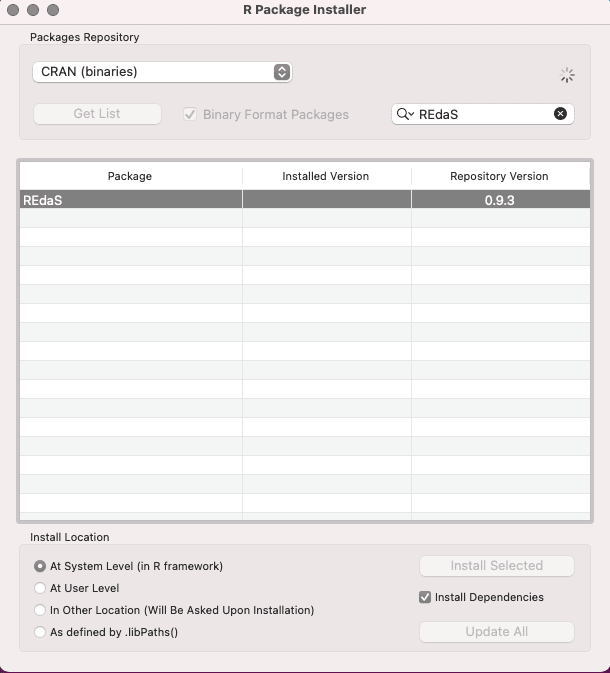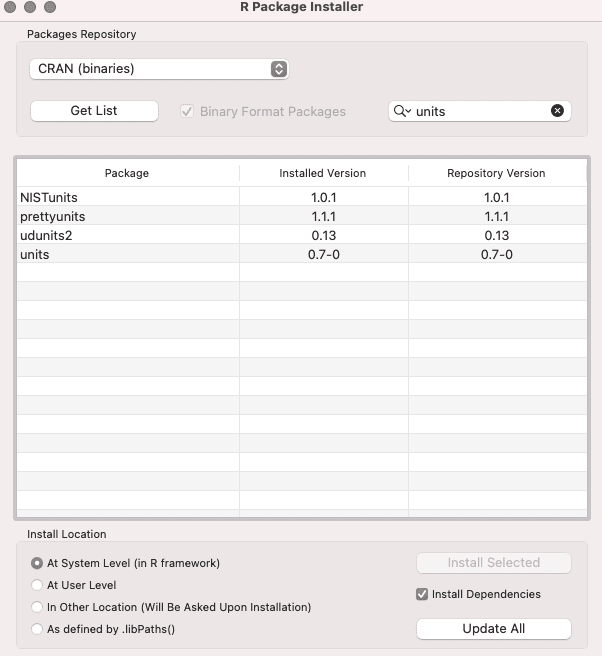# How to Convert Radians to Degrees in R

Radians and degrees are both units used for measuring angles. The circle is comprised of 2π radians, which is the equivalent of 360°. We often come across the scenarios in mathematical calculations in which we need to convert degrees to radians and radians to degrees. Fortunately, R provides packages that do this exactly. Let’s see how to do it.

## Convert Radians to Degrees in R

To convert radian values to degrees in R, use the rad2deg() function. The rad2deg() is a grid library’s function that calculates radians from degrees.

### Syntax

``rad2deg(rad)``

### Example

To work with the rad2deg() function, you must install the REdaS package in your system.The next step will be to create an R file and import the library using the following code.

``library(REdaS)``

Now, we can use the rad2deg() function to convert radian to degree values.

Take three-radian values and convert them to degrees.

``````library(REdaS)

The pi is a built-in constant in R.

#### Output

``````Loading required package: grid
 180
 179.9087
 180.0724``````

As you can see that the degree values of pi, 3.14, and 22/7 are 180, 179.9087, and 180.0724.

## Converting radians to degrees using units package

There is another third-party package called units that provides a function that will convert the radian values to degrees.The next step is to import the units library using the following code.

``library(units)``

Now, we can use the as_units() and set_units() functions to convert radian to degree values.

``````library(units)

pi_deg``````

#### Output

``180 [°]``

And we get the 180* value from the radian value.

Although it is always preferred to use the built-in or library-provided functions for conversion but, out of curiosity, you can create your own functions too. Let’s see how to create it.

``````rad2deg <- function(rad) {
}``````

Now, you can call the function and pass the values in the form of a radian, and it will return the value in degree.

``````rad2deg <- function(rad) {
}

``````

#### Output

`````` 180
 179.9087
 180.0724``````

Let’s create a custom function in R that converts degrees to radians.

``````deg2rad <- function(deg) {
(deg * pi) / (180)
}``````

Now, you can call the function and pass the values in the form of degree, and it will return the value in radian.

``````deg2rad <- function(deg) {
(deg * pi) / (180)
}

`````` 3.141593